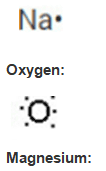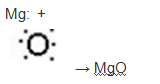• +91 9971497814
• info@interviewmaterial.com

# Chapter 3– Metals and Non-Metals Interview Questions Answers

### Related Subjects

Question 1 : Give an example of a metal which

(i) Is a liquid at room temperature?
(ii) Can be easily cut with a knife?
(iii) Is the best conductor of heat?
(iv) Is a poor conductor of heat?

(i) Is a liquid at room temperature?
(i) Mercury is the metal which is liquid at room temperature

(ii) Can be easily cut with a knife?
(ii) Sodium and potassium are the metals which can be cut with a knife

(iii) Is the best conductor of heat?
(iii) Silver is the best conductor of heat

(iv) Is a poor conductor of heat?
(iv) Mercury is poor conductor of heat.

Question 2 : Explain the meanings of malleable and ductile.

1. Metals which can be beaten to sheets are said to be malleable
2. Metals which can be drawn into thin wires are said to be ductile

Question 3 : Why is sodium kept immersed in kerosene oil?

Answer 3 : Sodium is a reactive metals, if kept open it will react with oxygen to explore and catch fire. Sodium metal is kept immersed in kerosene to prevent their reaction with oxygen, moisture and carbon dioxide of air.

Question 4 :
Write equations for the reactions of

(i) iron with steam
(ii) calcium and potassium with water

(i) Iron reacts with steam to form a magnetic oxide of Fe with the liberation of H2.

3Fe(s) + 4H2O(g) → Fe3O4(s)+ 4H2(g)

(ii) Calcium reacts with water to form calcium hydroxide and hydrogen.

Ca(s) + 2H2O(I) → Ca(OH)2(aq)+ H2(g)

Potassium reacts with cold water violently immediately with evolution of H2 which catches fire.

2K(s) + 2H2O(I) → 2KOH(aq) + 2H2(g)

Question 5 : Samples of four metals A, B, C and D were taken and added to the following solution one by one.

Answer 5 : The results obtained have been tabulated as follows

 Metal Iron(II) sulphate Copper(II) sulphate Zinc sulphate Silver Nitrate A No reaction Displacement – – B Displacement – – – C No reaction No reaction No reaction Displacement D No reaction No reaction No reaction No reaction
Use the Table above to answer the following questions about metals A, B, C and D.
1. Which is the most reactive metal?
2. What would you observe if B is added to a solution of Copper (II) sulphate?
3. Arrange the metals A, B, C and D in the order of decreasing reactivity.

(i) Metal B is the most reactive as it gives displacement reaction with iron (II) sulphate.
(ii) When metal B is added to copper (II) sulphate solution, a displacement reaction will take place because of which the blue colour of copper (II) sulphate solution will fade and a red-brown deposit of copper will be formed on metal B.
(iii)Metal B is the most reactive because it displaces iron from its salt solution. Metal A is less reactive because it displaces copper from its salt solution. Metal C is still less reactive because it can displace only silver from its salt solution and metal D is the least reactive because it cannot displace any metal from its salt solution. Hence, the decreasing order of reactivity of the metals is B > A > C > D.

Question 6 : Which gas is produced when dilute hydrochloric acid is added to a reactive metal? Write the chemical reaction when iron reacts with dilute H2SO4.

Answer 6 : Hydrogen gas is liberated when dilute HCl is added to a reactive metal.

Fe(s) + H2SO4(aq) → FeSO4(aq)+ H2(g)

Question 7 : What would you observe when zinc is added to a solution of iron (II) sulphate? Write the chemical reaction that takes place.

Answer 7 : Zinc is more reactive (more electro positive) than iron. Therefore Zinc displaces Iron from its salt solution. The colour of ferrous sulphate is pale green, which turns colourless

FeSO4 + Zn → ZnSO4 +Fe(s)

Light green     Zincsulphate(Colourless).

Question 8 :
(i) Write the electron-dot structures for sodium, oxygen and magnesium.
(ii) Show the formation of Na2O and MgO by the transfer of electrons.
(iii)What are the ions present in these compounds?

(i) Sodium:(ii) Formation of Magnesium oxide:
When magnesium reacts with oxygen, the magnesium atom transfers its two outermost electrons to an oxygen atom. By losing two electrons, the magnesium atoms form a magnesium ion (Mg2+) and by gaining two electrons, the oxygen atom forms an oxide ion (O2-).Formation of Sodium oxide:
Two sodium atoms transfer their 2 outermost electrons to an oxygen atom. By losing two electrons, the two sodium atoms form tow sodium ions (2Na+). And by gaining two electrons, the oxygen atom forms an oxide ion (O2-).

(iii) The ions present in sodium oxide compound (Na2O) are sodium ions (2Na+) and oxide ions (O2-).
The ions present in Magnesium oxide compound (MgO) are magnesium ions Mg2+ and oxide ions (O2-).

Question 9 : Why do ionic compounds have high melting points?

Answer 9 : Ionic compounds are the ones which has both positive and negative charges. Hence there will be strong force of attraction between them. This make expenditure of lot of heat to break this force of attraction hence ionic compounds have high melting points.

Question 10 :
Define the following terms.
(i) Mineral
(ii) Ore
(iii) Gangue

1. Minerals are compounds (also known as elements) which are found naturally in the earth’s crust. E.g. Alums, K2SO4.Al2(SO4)3.24H2O, etc.
2. Ores are minerals from which metal can be extracted Ex: Bauxite Al2O3.2H2 is the ore of Al, copper pyrite CuFeS2.All minerals are not considered as ores but all ores are also minerals.
3. Ores mined from the earth are naturally contaminated with sand, rocky materials. There are impurities present in the ore which are known as gangue.

Todays Deals### Chapter 3– Metals and Non-Metals Contributorskrishan

Name:
Email:

# Latest News# 9000 interview questions in different categories